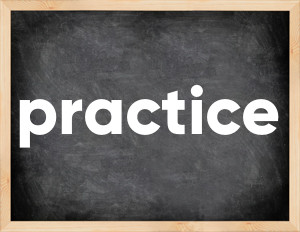# Practice past tenseThe English verb 'practice' is pronounced as [ˈpræktɪs].
Related to: regular verbs.
3 forms of verb practice: Infinitive (practice), Past Simple - (practiced), Past Participle - (practiced).

## Here are the past tense forms of the verb practice

👉 Forms of verb practice in future and past simple and past participle.
❓ What is the past tense of practice.

## Practice: Past, Present, and Participle Forms

Base Form Past Simple Past Participle
practice [ˈpræktɪs]

practiced [ˈpræktɪst]

practiced [ˈpræktɪst]

## What are the 2nd and 3rd forms of the verb practice?

🎓 What are the past simple, future simple, present perfect, past perfect, and future perfect forms of the base form (infinitive) 'practice'?

### Learn the three forms of the English verb 'practice'

• the first form (V1) is 'practice' used in present simple and future simple tenses.
• the second form (V2) is 'practiced' used in past simple tense.
• the third form (V3) is 'practiced' used in present perfect and past perfect tenses.

## What are the past tense and past participle of practice?

The past tense and past participle of practice are: practice in past simple is practiced, and past participle is practiced.

### What is the past tense of practice?

The past tense of the verb "practice" is "practiced", and the past participle is "practiced".

### Verb Tenses

Past simple — practice in past simple practiced (V2).
Future simple — practice in future simple is practice (will + V1).
Present Perfect — practice in present perfect tense is practiced (have/has + V3).
Past Perfect — practice in past perfect tense is practiced (had + V3).

### practice regular or irregular verb?

👉 Is 'practice' a regular or irregular verb? The verb 'practice' is regular verb.

## Examples of Verb practice in Sentences

•   We need to practice scales (Present Simple)
•   You practiced all day on 7 november (Past Simple)
•   He practices every day to get better at chess (Present Simple)
•   She has practiced singing for 5 years (Present Perfect)
•   Tim is busy, he's practicing the guitar (Present Continuous)
•   I'm forbidden to practice! (Present Simple)
•   We began to practice new techniques. (Past Simple)
•   My exam coming up, I really need to practice. (Present Simple)
•   It's hard to practice, because he distracts me (Present Simple)
•   He has practiced Spanish for a few years (Present Perfect)

Along with practice, words are popular override and swell.

Verbs by letter: , , , , , , , , , , , , , , , , , , , , , , , , .